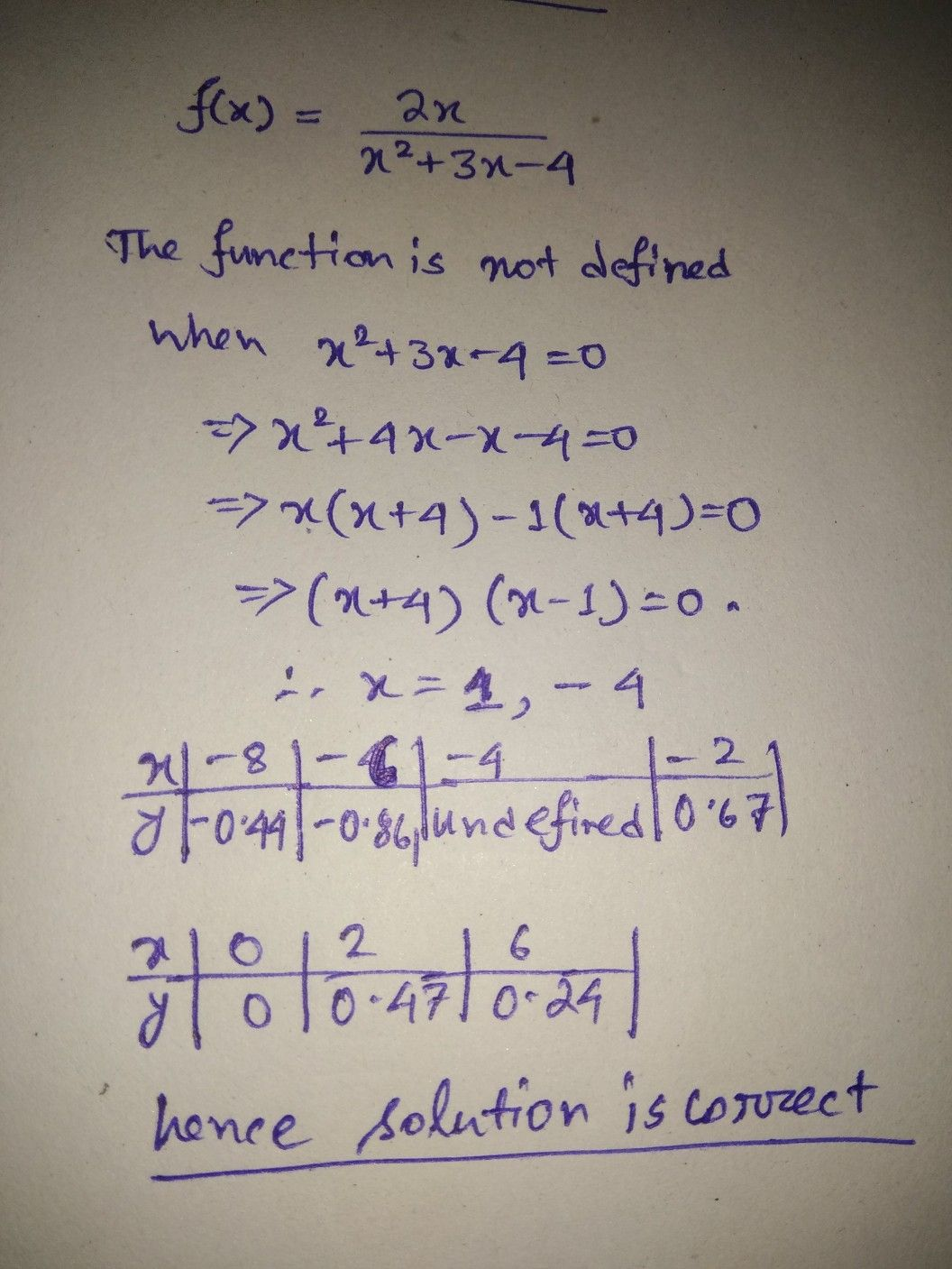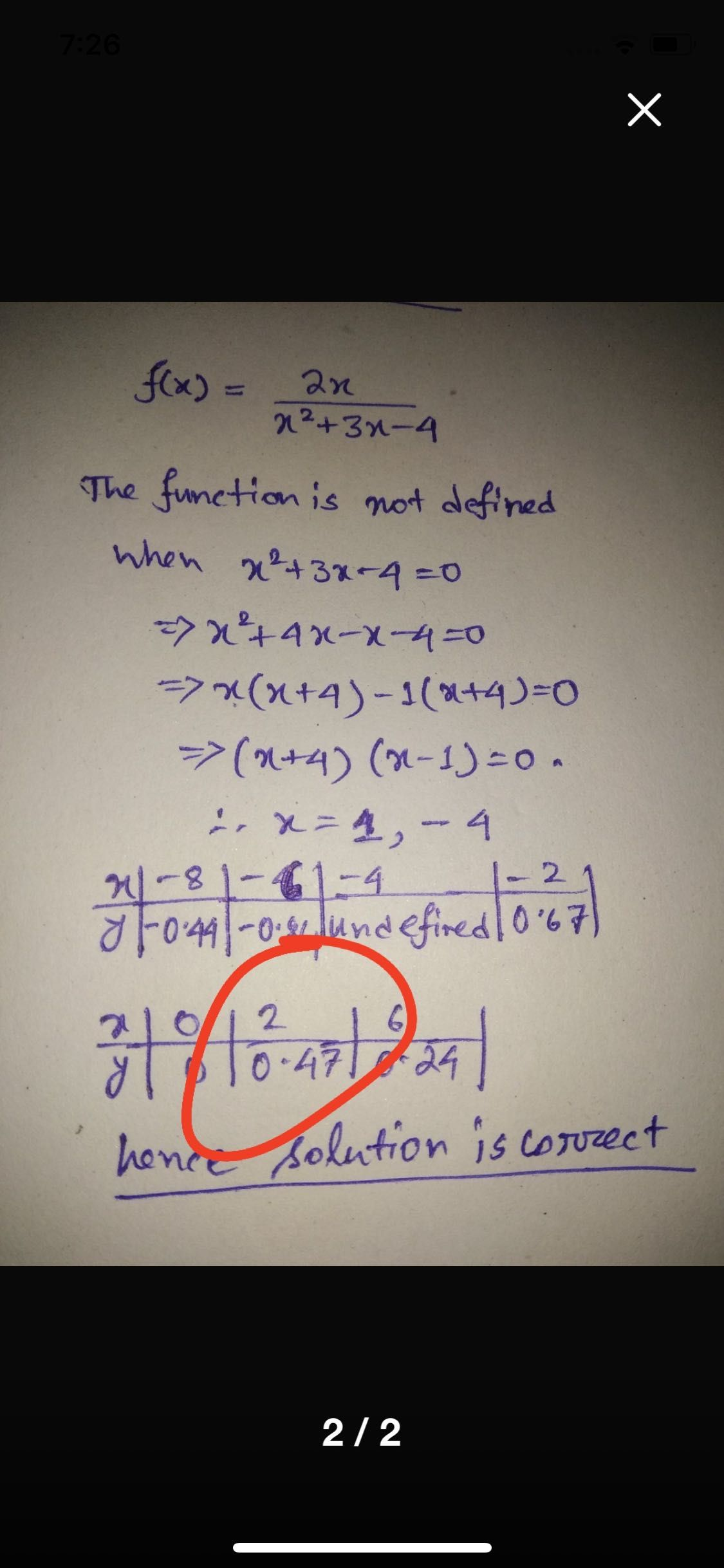Symbol
Problem$1$ Given the rational function $|\left(f\left(x\right)=lrgc\left(2x\right)\left(x^{n}2+3x-4\right)V$ solve for the domain and range; $X$ and $y$ intercepts; and vertical and horizontal asymptotes; intersection in HA and holes if there is any, of the given rational function. Complete the table below, graph and label the needed information. $\left(10$ points) $X$ $8$ $-6$ $-4$ $0$ $2$ $y$ $0.y4$ $-08b$ undefined $\dfrac {-2} {0.b7}$ $O$ $0.67$ $\dfrac {6} {0.2y}$
10th-13th grade
Other
Question content
Is my answer correct? Or is it wrong?:(
SolutionQanda teacher - CreationStudentCan I ask why did this become 0.47?